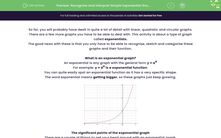# Recognise and Interpret Simple Exponential Graphs

In this worksheet, students will practise identifying and naming the significant points of exponential graphs.Key stage:  KS 4

Year:  GCSE

GCSE Subjects:   Maths

GCSE Boards:   AQA, Eduqas, OCR, Pearson Edexcel,

Curriculum topic:   Algebra, Graphs of Equations and Functions

Curriculum subtopic:   Graphs Graphs of Equations and Functions

Difficulty level:#### Worksheet Overview

So far, you will probably have dealt in quite a lot of detail with linear, quadratic and circular graphs.

There are a few more graphs you have to be able to deal with. This activity is about a type of graph called exponentials.

The good news with these is that you only have to be able to recognise, sketch and categorise these graphs and their function.

What is an exponential graph?

An exponential is any graph with the general form y = ax

For example: y = 4x is a exponential function.

You can quite easily spot an exponential function as it has a very specific shape.

The word exponential means getting bigger, so these graphs just keep growing.The significant points of the exponential graph

There are a couple of things to get your head around with an exponential graph.

Firstly, it has an asymptote on the x-axis. You can see from the graph above that the curve starts really close to the x–axis but never actually touches it.

Secondly, all exponential graphs cross at the point (0,1). Think logically about this - anything to the power of zero is always 1.

Let's try some questions now.

### What is EdPlace?

We're your National Curriculum aligned online education content provider helping each child succeed in English, maths and science from year 1 to GCSE. With an EdPlace account you’ll be able to track and measure progress, helping each child achieve their best. We build confidence and attainment by personalising each child’s learning at a level that suits them.

Get started